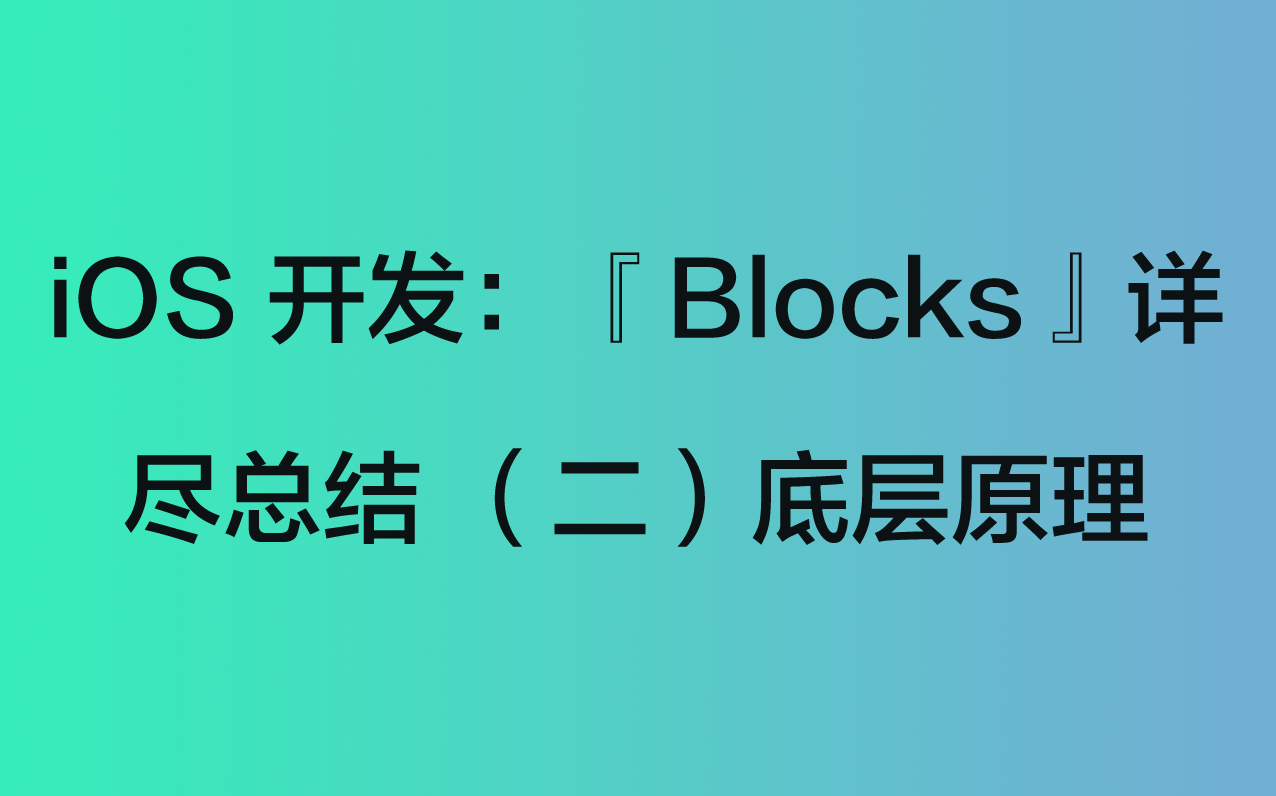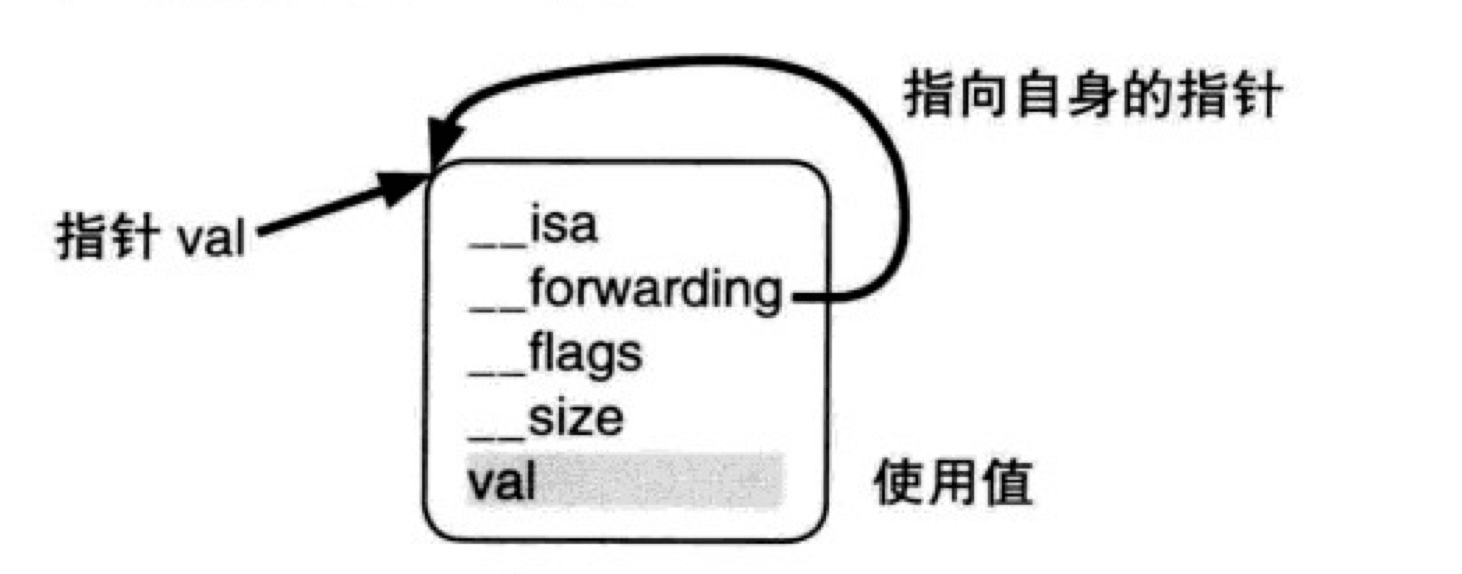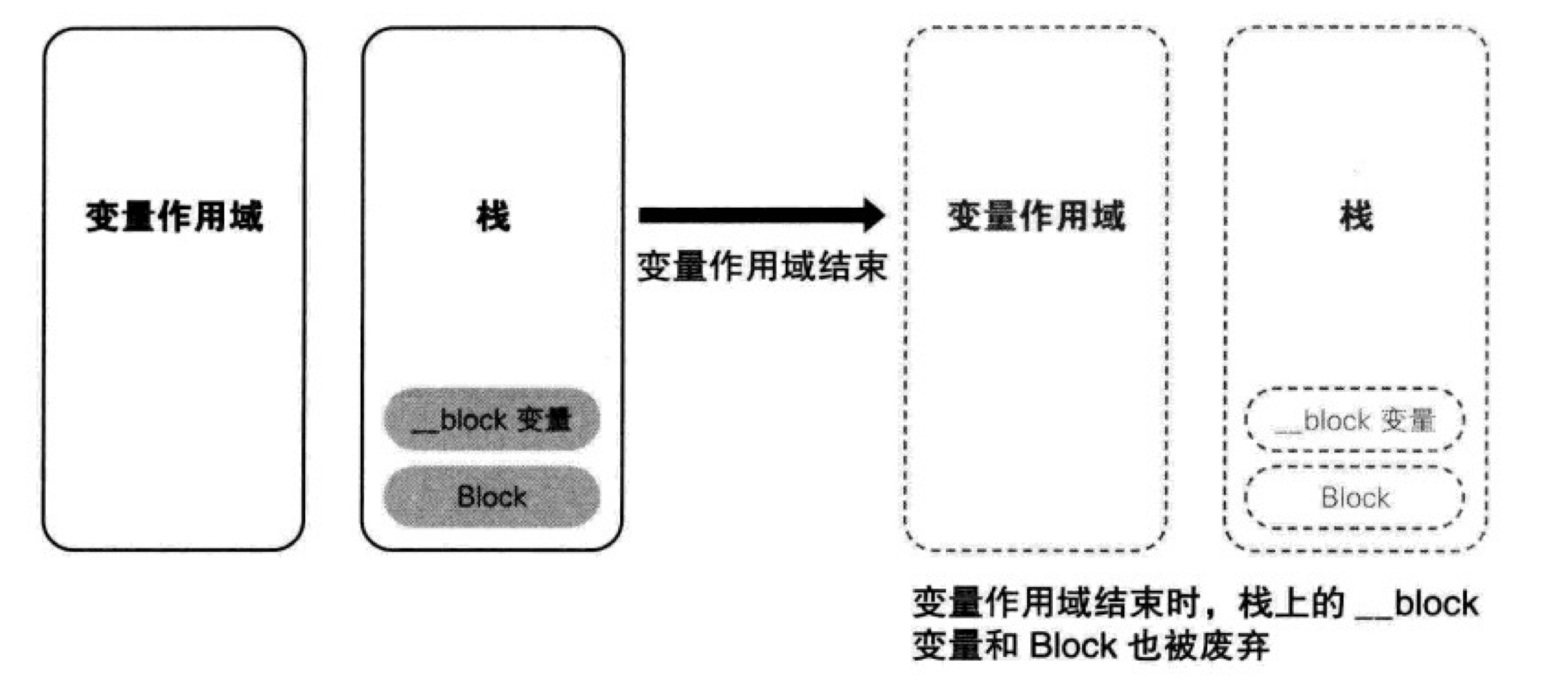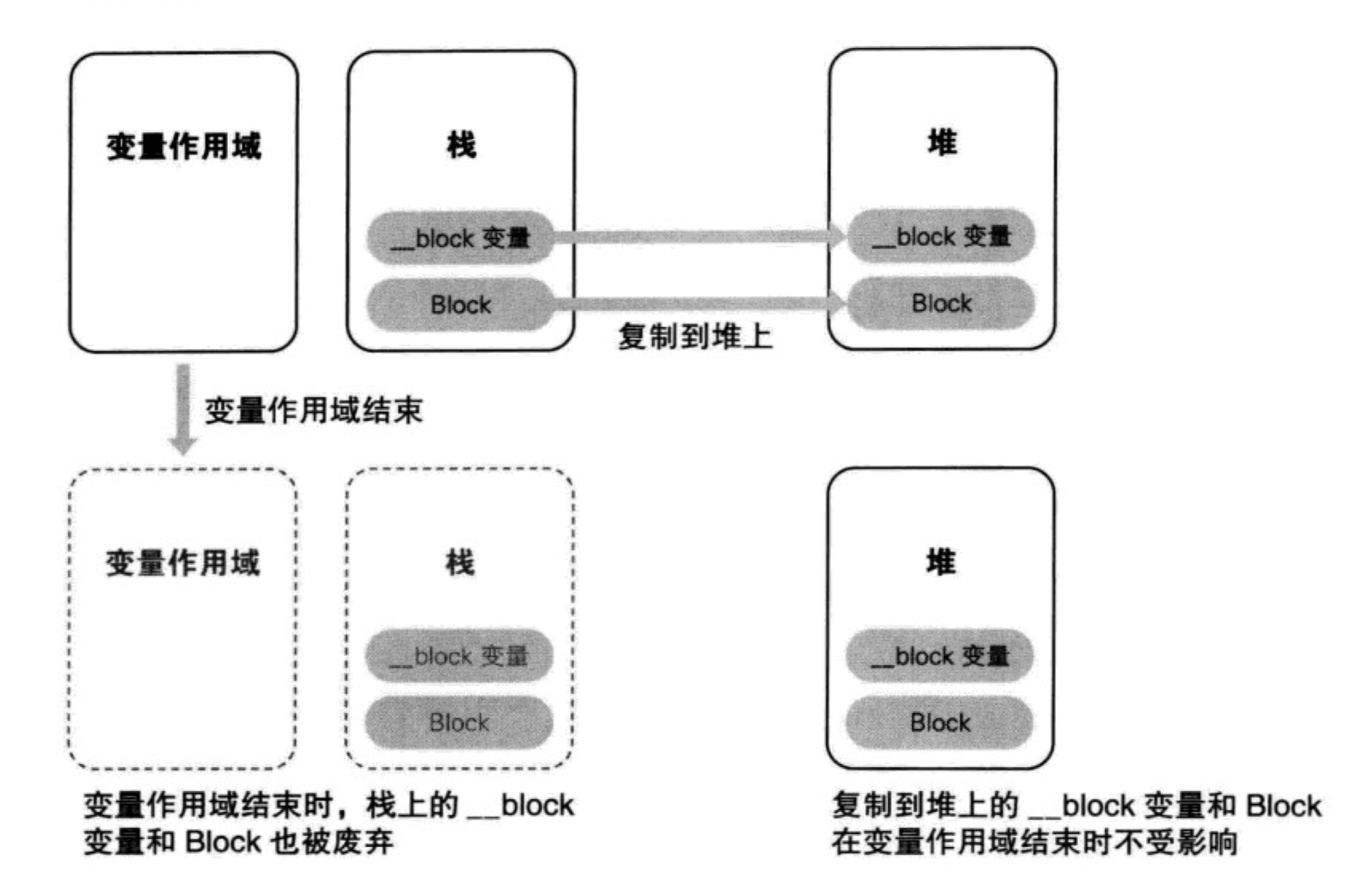# iOS 开发：「Blocks」详尽总结 （二）底层原理

20.832k 字  |  70 分钟

1. Blocks 的实质是什么？
2. Block 截获局部变量和特殊区域变量
3. Block 的存储区域
4. Block 的循环引用# 1. Blocks 的实质是什么？

## 1.1 Blocks 由 OC 转 C++ 源码方法

1. 在项目中添加 blocks.m 文件，并写好 block 的相关代码。
2. 打开「终端」，执行 cd XXX/XXX 命令，其中 XXX/XXX 为 block.m 所在的目录。
3. 继续执行clang -rewrite-objc block.m
4. 执行完命令之后，block.m 所在目录下就会生成一个 block.cpp 文件，这就是我们需要的 block 相关的 C++ 源码。

## 1.2 Blocks 源码概览

• 转换前 OC 代码：
int main () {
void (^myBlock)(void) = ^{
printf("myBlock\n");
};

myBlock();

return 0;
}
• 转换后 C++ 源码：
/* 包含 Block 实际函数指针的结构体 */
struct __block_impl {
void *isa;
int Flags;
int Reserved;        // 今后版本升级所需的区域大小
void *FuncPtr;      // 函数指针
};

/* Block 结构体 */
struct __main_block_impl_0 {
// impl：Block 的实际函数指针，指向包含 Block 主体部分的 __main_block_func_0 结构体
struct __block_impl impl;
// Desc：Desc 指针，指向包含 Block 附加信息的 __main_block_desc_0（） 结构体
struct __main_block_desc_0* Desc;
// __main_block_impl_0：Block 构造函数
__main_block_impl_0(void *fp, struct __main_block_desc_0 *desc, int flags=0) {
impl.isa = &_NSConcreteStackBlock;
impl.Flags = flags;
impl.FuncPtr = fp;
Desc = desc;
}
};

/* Block 主体部分结构体 */
static void __main_block_func_0(struct __main_block_impl_0 *__cself) {
printf("myBlock\n");
}

/* Block 附加信息结构体：包含今后版本升级所需区域大小，Block 的大小*/
static struct __main_block_desc_0 {
size_t reserved;        // 今后版本升级所需区域大小
size_t Block_size;    // Block 大小
} __main_block_desc_0_DATA = { 0, sizeof(struct __main_block_impl_0)};

/* main 函数 */
int main () {
void (*myBlock)(void) = ((void (*)())&__main_block_impl_0((void *)__main_block_func_0, &__main_block_desc_0_DATA));
((void (*)(__block_impl *))((__block_impl *)myBlock)->FuncPtr)((__block_impl *)myBlock);

return 0;
}

## 1.3 Block 结构体

/* Block 结构体 */
struct __main_block_impl_0 {
// impl：Block 的实际函数指针，指向包含 Block 主体部分的 __main_block_func_0 结构体
struct __block_impl impl;
// Desc：Desc 指针，指向包含 Block 附加信息的 __main_block_desc_0（） 结构体
struct __main_block_desc_0* Desc;
// __main_block_impl_0：Block 构造函数
__main_block_impl_0(void *fp, struct __main_block_desc_0 *desc, int flags=0) {
impl.isa = &_NSConcreteStackBlock;
impl.Flags = flags;
impl.FuncPtr = fp;
Desc = desc;
}
};

1. 成员变量 impl;
2. 成员变量 Desc 指针;
3. __main_block_impl_0 构造函数。

### 1.3.1 struct __block_impl impl 说明

/* 包含 Block 实际函数指针的结构体 */
struct __block_impl {
void *isa;               // 用于保存 Block 结构体的实例指针
int Flags;               // 标志位
int Reserved;        // 今后版本升级所需的区域大小
void *FuncPtr;      // 函数指针
};

### 1.3.2 struct __main_block_desc_0* Desc 说明

1. 今后版本升级所需区域大小： reserved 变量。
2. Block 大小：Block_size 变量。
/* Block 附加信息结构体：包含今后版本升级所需区域大小，Block 的大小*/
static struct __main_block_desc_0 {
size_t reserved;      // 今后版本升级所需区域大小
size_t Block_size;  // Block 大小
} __main_block_desc_0_DATA = { 0, sizeof(struct __main_block_impl_0)};

### 1.3.3 __main_block_impl_0 构造函数说明

__main_block_impl_0(void *fp, struct __main_block_desc_0 *desc, int flags=0) {
impl.isa = &_NSConcreteStackBlock;
impl.Flags = flags;
impl.FuncPtr = fp;
Desc = desc;
}

  void (*myBlock)(void) = ((void (*)())&__main_block_impl_0((void *)__main_block_func_0, &__main_block_desc_0_DATA));

struct __main_block_impl_0 temp = __main_block_impl_0(__main_block_func_0, &__main_block_desc_0_DATA);
struct __main_block_impl_0 myBlock = &temp;

1. 第一个参数：__main_block_func_0
- 其实就是 Block 对应的主体部分，可以看到下面关于 __main_block_func_0 结构体的定义 ，和 OC 代码中 ^{ printf("myBlock\n"); }; 部分具有相同的表达式。
- 这里参数中的 __cself 是指向 Block 的值的指针变量，相当于 OC 中的 self。

c++ /* Block 主体部分结构体 */ static void __main_block_func_0(struct __main_block_impl_0 *__cself) {      printf("myBlock\n"); }

1. 第二个参数：__main_block_desc_0_DATA__main_block_desc_0_DATA 包含该 Block 的相关信息。
我们再来结合之前的 __main_block_impl_0 结构体定义。
__main_block_impl_0 结构体（Block 结构体）可以表述为：

struct __main_block_impl_0 {
void *isa;               // 用于保存 Block 结构体的实例指针
int Flags;               // 标志位
int Reserved;        // 今后版本升级所需的区域大小
void *FuncPtr;      // 函数指针
struct __main_block_desc_0* Desc;      // Desc：Desc 指针
};

__main_block_impl_0 构造函数可以表述为：

impl.isa = &_NSConcreteStackBlock;    // isa 保存 Block 结构体实例
impl.Flags = 0;        // 标志位赋值
impl.FuncPtr = __main_block_func_0;    // FuncPtr 保存 Block 结构体的主体部分
Desc = &__main_block_desc_0_DATA;    // Desc 保存 Block 结构体的附加信息

## 1.4 Block 实质总结

__main_block_impl_0 结构体（Block 结构体）相当于 Objective-C 类对象的结构体，isa 指针保存的是所属类的结构体的实例的指针。_NSConcreteStackBlock 相当于 Block 的结构体实例。对象 impl.isa = &_NSConcreteStackBlock; 语句中，将 Block 结构体的指针赋值给其成员变量 isa，相当于 Block 结构体的成员变量 保存了 Block 结构体的指针，这里和 Objective-C 中的对象处理方式是一致的。

也就是说明： Block 的实质就是对象。
Block 跟其他所有的 NSObject 一样，都是对象。果不其然，万物皆对象，古人诚不欺我。

# 2. Block 截获局部变量和特殊区域变量

## 2.1 Blcok 截获局部变量的实质

// 使用 Blocks 截获局部变量值
- (void)useBlockInterceptLocalVariables {
int a = 10, b = 20;

void (^myLocalBlock)(void) = ^{
printf("a = %d, b = %d\n",a, b);
};

myLocalBlock();    // 输出结果：a = 10, b = 20

a = 20;
b = 30;

myLocalBlock();    // 输出结果：a = 10, b = 20
}

struct __main_block_impl_0 {
struct __block_impl impl;
struct __main_block_desc_0* Desc;
int a;
int b;
__main_block_impl_0(void *fp, struct __main_block_desc_0 *desc, int _a, int _b, int flags=0) : a(_a), b(_b) {
impl.isa = &_NSConcreteStackBlock;
impl.Flags = flags;
impl.FuncPtr = fp;
Desc = desc;
}
};

static void __main_block_func_0(struct __main_block_impl_0 *__cself) {
int a = __cself->a; // bound by copy
int b = __cself->b; // bound by copy

printf("a = %d, b = %d\n",a, b);
}

static struct __main_block_desc_0 {
size_t reserved;
size_t Block_size;
} __main_block_desc_0_DATA = { 0, sizeof(struct __main_block_impl_0)};

int main () {
int a = 10, b = 20;

void (*myLocalBlock)(void) = ((void (*)())&__main_block_impl_0((void *)__main_block_func_0, &__main_block_desc_0_DATA, a, b));
((void (*)(__block_impl *))((__block_impl *)myLocalBlock)->FuncPtr)((__block_impl *)myLocalBlock);

a = 20;
b = 30;

((void (*)(__block_impl *))((__block_impl *)myLocalBlock)->FuncPtr)((__block_impl *)myLocalBlock);
}
1. 可以看到 __main_block_impl_0 结构体（Block 结构体）中多了两个成员变量 ab，这两个变量就是 Block 截获的局部变量。 ab 的值来自与 __main_block_impl_0 构造函数中传入的值。

struct main_block_impl_0 {
struct __block_impl impl;
struct
main_block_desc_0 Desc;
int a;    // 增加的成员变量 a
int b;    // 增加的成员变量 b
__main_block_impl_0(void
fp, struct __main_block_desc_0 *desc, int _a, int _b, int flags=0) : a(_a), b(_b) {
impl.isa = &_NSConcreteStackBlock;
impl.Flags = flags;
impl.FuncPtr = fp;
Desc = desc;
}
};



2. 还可以看出 __main_block_func_0（保存 Block 主体部分的结构体）中，变量 a、b 的值使用的 cself 获取的值。
而 
cself->a、__cself->b 是通过值传递的方式传入进来的，而不是通过指针传递。这也就说明了 a、b 只是 Block 内部的变量，改变 Block 外部的局部变量值，并不能改变 Block 内部的变量值。

    static void __main_block_func_0(struct __main_block_impl_0 *__cself) {
int a = __cself->a; // bound by copy
int b = __cself->b; // bound by copy
printf("a = %d, b = %d\n",a, b);
}

int a = 10, b = 20;

void (^myLocalBlock)(void) = ^{
a = 20;
b = 30;

printf("a = %d, b = %d\n",a, b);
};

myLocalBlock();## 2.2 使用 __block 说明符更改局部变量值

// 使用 __block 说明符修饰，更改局部变量值
- (void)useBlockQualifierChangeLocalVariables {
__block int a = 10, b = 20;

void (^myLocalBlock)(void) = ^{
a = 20;
b = 30;

printf("a = %d, b = %d\n",a, b);    // 输出结果：a = 20, b = 30
};

myLocalBlock();
}

struct __Block_byref_a_0 {
void *__isa;
__Block_byref_a_0 *__forwarding;
int __flags;
int __size;
int a;
};

struct __Block_byref_b_1 {
void *__isa;
__Block_byref_b_1 *__forwarding;
int __flags;
int __size;
int b;
};

struct __main_block_impl_0 {
struct __block_impl impl;
struct __main_block_desc_0* Desc;
__Block_byref_a_0 *a; // by ref
__Block_byref_b_1 *b; // by ref
__main_block_impl_0(void *fp, struct __main_block_desc_0 *desc, __Block_byref_a_0 *_a, __Block_byref_b_1 *_b, int flags=0) : a(_a->__forwarding), b(_b->__forwarding) {
impl.isa = &_NSConcreteStackBlock;
impl.Flags = flags;
impl.FuncPtr = fp;
Desc = desc;
}
};

static void __main_block_func_0(struct __main_block_impl_0 *__cself) {
__Block_byref_a_0 *a = __cself->a; // bound by ref
__Block_byref_b_1 *b = __cself->b; // bound by ref

(a->__forwarding->a) = 20;
(b->__forwarding->b) = 30;

printf("a = %d, b = %d\n",(a->__forwarding->a), (b->__forwarding->b));
}

static void __main_block_copy_0(struct __main_block_impl_0*dst, struct __main_block_impl_0*src) {_Block_object_assign((void*)&dst->a, (void*)src->a, 8/*BLOCK_FIELD_IS_BYREF*/);_Block_object_assign((void*)&dst->b, (void*)src->b, 8/*BLOCK_FIELD_IS_BYREF*/);}

static void __main_block_dispose_0(struct __main_block_impl_0*src) {_Block_object_dispose((void*)src->a, 8/*BLOCK_FIELD_IS_BYREF*/);_Block_object_dispose((void*)src->b, 8/*BLOCK_FIELD_IS_BYREF*/);}

static struct __main_block_desc_0 {
size_t reserved;
size_t Block_size;
void (*copy)(struct __main_block_impl_0*, struct __main_block_impl_0*);
void (*dispose)(struct __main_block_impl_0*);
} __main_block_desc_0_DATA = { 0, sizeof(struct __main_block_impl_0), __main_block_copy_0, __main_block_dispose_0};

int main() {
__attribute__((__blocks__(byref))) __Block_byref_a_0 a = {(void*)0,(__Block_byref_a_0 *)&a, 0, sizeof(__Block_byref_a_0), 10};
__Block_byref_b_1 b = {(void*)0,(__Block_byref_b_1 *)&b, 0, sizeof(__Block_byref_b_1), 20};

void (*myLocalBlock)(void) = ((void (*)())&__main_block_impl_0((void *)__main_block_func_0, &__main_block_desc_0_DATA, (__Block_byref_a_0 *)&a, (__Block_byref_b_1 *)&b, 570425344));
((void (*)(__block_impl *))((__block_impl *)myLocalBlock)->FuncPtr)((__block_impl *)myLocalBlock);

return 0;
}

struct __main_block_impl_0 {
struct __block_impl impl;
struct __main_block_desc_0* Desc;
__Block_byref_a_0 *a; // by ref
__Block_byref_b_1 *b; // by ref
__main_block_impl_0(void *fp, struct __main_block_desc_0 *desc, __Block_byref_a_0 *_a, __Block_byref_b_1 *_b, int flags=0) : a(_a->__forwarding), b(_b->__forwarding) {
impl.isa = &_NSConcreteStackBlock;
impl.Flags = flags;
impl.FuncPtr = fp;
Desc = desc;
}
};

__Block_byref_a_0、__Block_byref_b_1 类型的结构体声明如下：


struct __Block_byref_a_0 {
void *__isa;
__Block_byref_a_0 *__forwarding;
int __flags;
int __size;
int a;
};

struct __Block_byref_b_1 {
void *__isa;
__Block_byref_b_1 *__forwarding;
int __flags;
int __size;
int b;
};

1. __isa：标识对象类的 isa 实例变量
2. __forwarding：传入变量的地址
3. __flags：标志位
4. __size：结构体大小
5. a：存放实变量 a 实际的值，相当于原局部变量的成员变量（和之前不加__block修饰符的时候一致）。

__Block_byref_a_0 a = {
(void*)0,
(__Block_byref_a_0 *)&a,
0,
sizeof(__Block_byref_a_0),
10
};

__Block_byref_b_1 b = {
0,
&b,
0,
sizeof(__Block_byref_b_1),
20
};static void __main_block_func_0(struct __main_block_impl_0 *__cself) {
__Block_byref_a_0 *a = __cself->a; // bound by ref
__Block_byref_b_1 *b = __cself->b; // bound by ref

(a->__forwarding->a) = 20;
(b->__forwarding->b) = 30;

printf("a = %d, b = %d\n",(a->__forwarding->a), (b->__forwarding->b));
}

## 2.3 更改特殊区域变量值

• OC 代码：
int global_val = 10; // 全局变量
static int static_global_val = 20; // 静态全局变量

int main() {
static int static_val = 30; // 静态局部变量

void (^myLocalBlock)(void) = ^{
global_val *= 1;
static_global_val *= 2;
static_val *= 3;

printf("static_val = %d, static_global_val = %d, global_val = %d\n",static_val, static_global_val, static_val);
};

myLocalBlock();

return 0;
}

• C++ 代码：
int global_val = 10;
static int static_global_val = 20;

struct __main_block_impl_0 {
struct __block_impl impl;
struct __main_block_desc_0* Desc;
int *static_val;
__main_block_impl_0(void *fp, struct __main_block_desc_0 *desc, int *_static_val, int flags=0) : static_val(_static_val) {
impl.isa = &_NSConcreteStackBlock;
impl.Flags = flags;
impl.FuncPtr = fp;
Desc = desc;
}
};

static void __main_block_func_0(struct __main_block_impl_0 *__cself) {
int *static_val = __cself->static_val; // bound by copy
global_val *= 1;
static_global_val *= 2;
(*static_val) *= 3;

printf("static_val = %d, static_global_val = %d, global_val = %d\n",(*static_val), static_global_val, (*static_val));
}

static struct __main_block_desc_0 {
size_t reserved;
size_t Block_size;
} __main_block_desc_0_DATA = { 0, sizeof(struct __main_block_impl_0)};

int main() {
static int static_val = 30;

void (*myLocalBlock)(void) = ((void (*)())&__main_block_impl_0((void *)__main_block_func_0, &__main_block_desc_0_DATA, &static_val));
((void (*)(__block_impl *))((__block_impl *)myLocalBlock)->FuncPtr)((__block_impl *)myLocalBlock);

return 0;

}


int global_val = 10;
static int static_global_val = 20;

struct __main_block_impl_0 {
struct __block_impl impl;
struct __main_block_desc_0* Desc;
int *static_val;
__main_block_impl_0(void *fp, struct __main_block_desc_0 *desc, int *_static_val, int flags=0) : static_val(_static_val) {
impl.isa = &_NSConcreteStackBlock;
impl.Flags = flags;
impl.FuncPtr = fp;
Desc = desc;
}
};

static void __main_block_func_0(struct __main_block_impl_0 *__cself) {
int *static_val = __cself->static_val; // bound by copy
global_val *= 1;
static_global_val *= 2;
(*static_val) *= 3;

printf("static_val = %d, static_global_val = %d, global_val = %d\n",(*static_val), static_global_val, (*static_val));
}

# 3. Block 的存储区域

## 3.1 _NSConcreteGlobalBlock

1. 记述全局变量的地方，使用 Block 语法时；
2. Block 语法的表达式中没有截获的自动变量时。

NSConcreteGlobalBlock 类的 Block 存储在「程序的数据区域」。因为存放在程序的数据区域，所以即使在变量的作用域外，也可以通过指针安全的使用。

• 记述全局变量的地方，使用 Block 语法示例代码：
void (^myGlobalBlock)(void) = ^{
printf("GlobalBlock\n");
};

int main() {
myGlobalBlock();

return 0;
}

## 3.2 _NSConcreteStackBlock

NSConcreteStackBlock 类的 Block 存储在「栈区」的。如果其所属的变量作用域结束，则该 Block 就会被废弃。如果 Block 使用了 block 变量，则当 block 变量的作用域结束，则 __block 变量同样被废弃。## 3.3 _NSConcreteMallocBlock## 3.4 Block 的自动拷贝和手动拷贝

### 3.4.1 Block 的自动拷贝

1. 将 Block 作为函数返回值返回时，会自动拷贝；
2. 向方法或函数的参数中传递 Block 时，使用以下两种方法的情况下，会进行自动拷贝，否则就需要手动拷贝：
1. Cocoa 框架的方法且方法名中含有 usingBlock 等时；
2. Grand Central Dispatch（GCD） 的 API。

### 3.4.2 Block 的手动拷贝

Block 类  存储区域 拷贝效果
_NSConcreteStackBlock  栈区 从栈拷贝到堆
_NSConcreteGlobalBlock  程序的数据区域 不做改变
_NSConcreteMallocBlock  堆区 引用计数增加

## 3.5 __block 变量的拷贝

__block 变量的配置存储区域 Block 从栈复制到堆时的影响

# 4. Block 的循环引用

/* —————— retainCycleBlcok.m —————— */
#import
#import "Person.h"

int main() {
Person *person = [[Person alloc] init];
person.blk = ^{
NSLog(@"%@",person);
};

return 0;
}

/* —————— Person.h —————— */
#import

typedef void(^myBlock)(void);

@interface Person : NSObject
@property (nonatomic, copy) myBlock blk;
@end

/* —————— Person.m —————— */
#import "Person.h"

@implementation Person

@end

struct __main_block_impl_0 {
struct __block_impl impl;
struct __main_block_desc_0* Desc;
Person *person;
__main_block_impl_0(void *fp, struct __main_block_desc_0 *desc, Person *_person, int flags=0) : person(_person) {
impl.isa = &_NSConcreteStackBlock;
impl.Flags = flags;
impl.FuncPtr = fp;
Desc = desc;
}
};

static void __main_block_func_0(struct __main_block_impl_0 *__cself) {
Person *person = __cself->person; // bound by copy

NSLog((NSString *)&__NSConstantStringImpl__var_folders_ct_0dyw1pvj6k16t5z8t0j0_ghw0000gn_T_retainCycleBlcok_8957e0_mi_0,person);
}

## 4.1 ARC 下，通过 __weak 修饰符来消除循环引用

int main() {
Person *person = [[Person alloc] init];
__weak typeof(person) weakPerson = person;

person.blk = ^{
NSLog(@"%@",weakPerson);
};

return 0;
}

struct __main_block_impl_0 {
struct __block_impl impl;
struct __main_block_desc_0* Desc;
Person *__weak weakPerson;
__main_block_impl_0(void *fp, struct __main_block_desc_0 *desc, Person *__weak _weakPerson, int flags=0) : weakPerson(_weakPerson) {
impl.isa = &_NSConcreteStackBlock;
impl.Flags = flags;
impl.FuncPtr = fp;
Desc = desc;
}
};

static void __main_block_func_0(struct __main_block_impl_0 *__cself) {
Person *__weak weakPerson = __cself->weakPerson; // bound by copy

NSLog((NSString *)&__NSConstantStringImpl__var_folders_ct_0dyw1pvj6k16t5z8t0j0_ghw0000gn_T_retainCycleBlcok_447367_mi_0,weakPerson);
}

## 4.2 MRC 下，通过 __block 修饰符来消除循环引用

MRC 下，是不支持 weak 修饰符的。我们可以通过 block 来消除循环引用。

int main() {
Person *person = [[Person alloc] init];
__block typeof(person) blockPerson = person;

person.blk = ^{
NSLog(@"%@", blockPerson);
};

return 0;
}

struct __main_block_impl_0 {
struct __block_impl impl;
struct __main_block_desc_0* Desc;
__Block_byref_blockPerson_0 *blockPerson; // by ref
__main_block_impl_0(void *fp, struct __main_block_desc_0 *desc, __Block_byref_blockPerson_0 *_blockPerson, int flags=0) : blockPerson(_blockPerson->__forwarding) {
impl.isa = &_NSConcreteStackBlock;
impl.Flags = flags;
impl.FuncPtr = fp;
Desc = desc;
}
};

static void __main_block_func_0(struct __main_block_impl_0 *__cself) {
__Block_byref_blockPerson_0 *blockPerson = __cself->blockPerson; // bound by ref

NSLog((NSString *)&__NSConstantStringImpl__var_folders_ct_0dyw1pvj6k16t5z8t0j0_ghw0000gn_T_retainCycleBlcok_536cd4_mi_0,(blockPerson->__forwarding->blockPerson));
}

# 评论（没有评论）# 程序员充电站微信支付支付宝